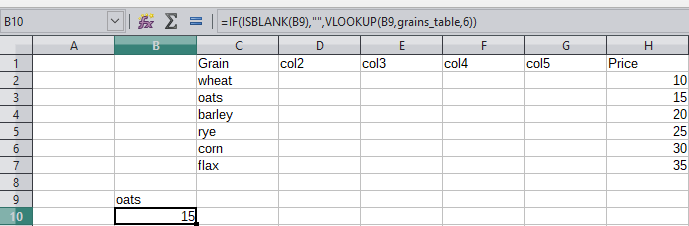Getting err 504 with complex function

I am using a file created in excel and when I go to use the file in calc I am getting err 504 from many formulas. The formulas use info contained inside sheets and tables in the file.

=IF(ISBLANK(B9),"",VLOOKUP(B9,grains_table[#all],6))

Welcome to the forum, but …
Do you expect someone here to know what the grains_table is supposed to mean?

The other person who answered provided a very useful answer, maybe i didnt give the best information but it was enough for someone else to figure out. They understood from the formula that ‘grains_table’ was a defined range/array.

I also guessed this. In fact I had already prepared a working example. However grains_table[#all] isn’t correct/meaningful synatx in Calc, I would still want to know in what way this kind of subexpression can be used in Excel.
BTW: I much appreciate “the other person” who is @jimk .
(Better information is better than probably sufficient information.)

Remove [#all] from the formula and make sure grains_table is defined.

To see how this works, start with an empty spreadsheet. Put table data in C1 to H7 such as shown in the image below. Then go to Data → Define Range and define the name grains_table as \$Sheet1.\$C\$2:\$H\$7. Enter “oats” (without quotes) in B9 and the following formula in B10.

=IF(ISBLANK(B9);"";VLOOKUP(B9;grains_table;6))